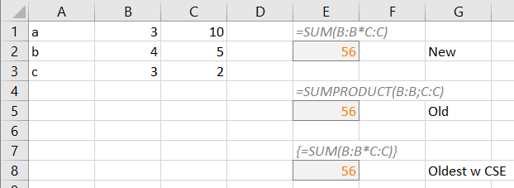# SUM(B:B*C:C)

A "small" one is that SUM now can do subcalculations before performing the final sum. Eg the rows in an invoice with count and price can now be done like =SUM(B:B*C:C) and result in the sum of b1*c1 + b2*c2 + b3*c3 etc

The possibility has actually been around for a long time but quite unknown since it in older versions is needed to enter the formula with Control+Shift+Enter.

Summing products became easier by the added function SUMPRODUCT but still most users append a helper column doing the calculation, row by row, and finally summarize the extra column.• SUM
• SUMPRODUCT
• Control+Shift+Enter (CSE)